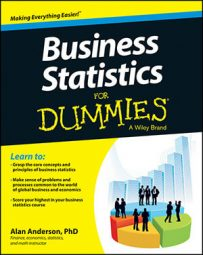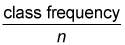A frequency distribution shows the number of elements in a data set that belong to each class. In a relative frequency distribution, the value assigned to each class is the proportion of the total data set that belongs in the class.

For example, suppose that a frequency distribution is based on a sample of 200 supermarkets. It turns out that 50 of these supermarkets charge a price between \$8.00 and \$8.99 for a pound of coffee. In a relative frequency distribution, the number assigned to this class would be 0.25 (50/200). In other words, that's 25 percent of the total.

Here's a handy formula for calculating the relative frequency of a class:Class frequency refers to the number of observations in each class; n represents the total number of observations in the entire data set. For the supermarket example, the total number of observations is 200.

The relative frequency may be expressed as a proportion (fraction) of the total or as a percentage of the total. For example, the following table shows the frequency distribution of gas prices at 20 different stations.

Frequency Distribution of Prices for 20 Gas Stations
Gas Prices (\$/Gallon) Number of Gas Stations
\$3.50–\$3.74 6
\$3.75–\$3.99 4
\$4.00–\$4.24 5
\$4.25–\$4.49 5

Based on this information, you can use the relative frequency formula to create the next table, which shows the relative frequency of the prices in each class, as both a fraction and a percentage.

Relative Frequencies for Gas Station Prices
Gas Prices (\$/Gallon) Number of Gas Stations Relative Frequency
(fraction)
Relative Frequency
(percent)
\$3.50–\$3.74 6 6/20 = 0.30 30%
\$3.75–\$3.99 4 4/20 = 0.20 20%
\$4.00–\$4.24 5 5/20 = 0.25 25%
\$4.25–\$4.49 5 5/20 = 0.25 25%

With a sample size of 20 gas stations, the relative frequency of each class equals the actual number of gas stations divided by 20. The result is then expressed as either a fraction or a percentage. For example, you calculate the relative frequency of prices between \$3.50 and \$3.74 as 6/20 to get 0.30 (30 percent). Similarly, the relative frequency of prices between \$3.75 and \$3.99 equals 4/20 = 0.20 = 20 percent.

One of the advantages of using a relative frequency distribution is that you can compare data sets that don't necessarily contain an equal number of observations. For example, suppose that a researcher is interested in comparing the distribution of gas prices in New York and Connecticut. Because New York has a much larger population, it also has many more gas stations. The researcher decides to choose 1 percent of the gas stations in New York and 1 percent of the gas stations in Connecticut for the sample. This turns out to be 800 in New York and 200 in Connecticut. The researcher puts together a frequency distribution as shown in the next table.

Frequency Distribution of Gas Prices in New York and Connecticut
Price New York Gas Stations Connecticut Gas Stations
\$3.00–\$3.49 210 48
\$3.50–\$3.99 420 96
\$4.00–\$4.49 170 56

Based on this frequency distribution, it's awkward to compare the distribution of prices in the two states. By converting this data into a relative frequency distribution, the comparison is greatly simplified, as seen in the final table.

Relative Frequency Distribution of Gas Prices in New York and Connecticut
Price New York Gas Stations Relative Frequency Connecticut Gas Stations Relative Frequency
\$3.00–\$3.49 210 210/800 = 0.2625 48 48/200 = 0.2400
\$3.50–\$3.99 420 420/800 = 0.5250 96 96/200 = 0.4800
\$4.00–\$4.49 170 170/800 = 0.2125 56 56/200 = 0.2800

The results show that the distribution of gas prices in the two states is nearly identical. Roughly 25 percent of the gas stations in each state charge a price between \$3.00 and \$3.49; about 50 percent charge a price between \$3.50 and \$3.99; and about 25 percent charge a price between \$4.00 and \$4.49.